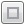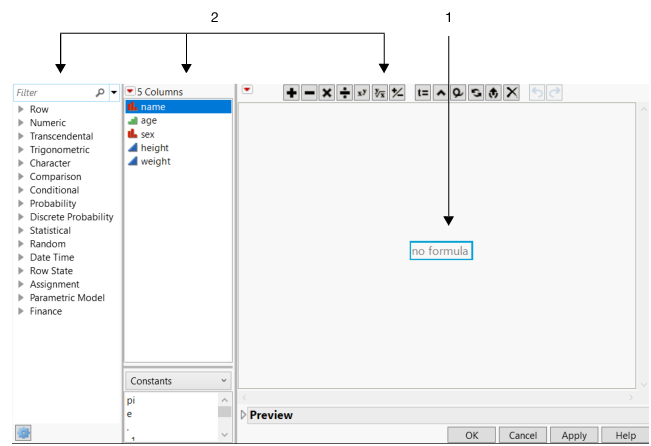Using JMP > Formula Editor > Create a Formula
Publication date: 08/13/2020

# Create a Formula

A formula is an expression stored in a column that performs operations in order to insert values into that column. Formulas can perform mathematical operations, such as addition and multiplication, or they can compare column values or join values by referring to other areas in the same data table. Formulas can consist of any JMP Scripting Language (JSL) command. Once you insert a formula into a column, the column is locked: its values can be edited only by changing or removing the formula.

There are three basic steps to building a formula:

1. Open the Formula Editor by right-clicking the column name to which you want to apply the formula and selecting Formula.

or

Double-click the column name to which you want to apply the formula, select Formula from the Column Properties menu, and then click Edit Formula.

In the Formula Editor, the empty formula is selected (highlighted in blue). You can start typing or select a column or function.

When you type, a small text editor window appears, which enables you to edit the formula. You can click the Maximize editor buttonif you need more room. Long formulas open in the maximized editor by default.

When you select a column or function, that item is added to the selected blue box.

You can also drag a column from the Columns list into the selected box.

Note: An element is selected when there is a blue outline around it. All terms within the smallest nesting box relative to the place that you clicked become selected. The subsequent actions apply to those combined elements.

2. Add expressions, functions, and terms. Then they appear in the highlighted blue box. The following sections in this chapter provide detailed instructions on how to add constants, elements, operators, and functions.

Figure 7.2 Building a FormulaSee Use Basic Formula Editor Features, for an example of how to use the Formula Editor.

Notes:

In a formula, when you reference a column using value labels, place your cursor over the value label to see the actual data value.

The Formula Editor recognizes the Excluded state and removes those rows from calculations. Here’s an example using the Col Maximum() function:

`Col Maximum( :height, :sex, Excluded( Row State() ) )`

Whitespace in a formula is automatically removed by JMP.

You can also use the Formula Editor to define a custom format. For example, you might want to convert a number from inches to centimeters using the calculation Char( :height * 2.54 ) || " cm". Realize that the underlying data, which you see when double-clicking a data table cell, would still be the value of :height.

To move the cursor from one field to another in a formula, select the field and press an arrow key.

For aggregated functions such as Col Mean, the formula might evaluate faster if you separate the formula into two columns. One column should contain an expression that represents the target for the aggregated function. The second column should contain the aggregated function itself referencing the new target column. Here’s an example of the original formula:

`:Y - Col Mean( If( :Status == "nonsmoker", :Y, . ), :Cycle )`

In a new Y2 column, create this formula:

`If( :Status == "nonsmoker",`
`	:Y,`
`	.`
`)`

In another column, create this formula:

`:Y - Col Mean( :Name( :Y2, :Cycle )`